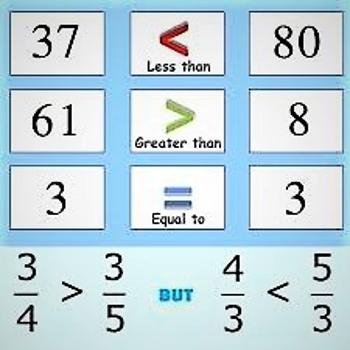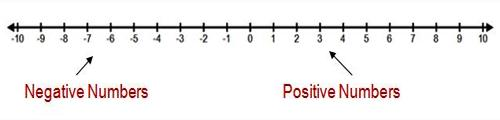Comparing Integers

If there are two numbers we can compare them.  One number is either greater than, less than or equal to the other number.

If the first number has a higher count than the second number, it is greater than the second number. The symbol “>” is used to mean greater than.  In this example, we could say either “15 is greater than 9” or “15 > 9”.  The greater than symbol can be remembered because the larger open end is near the larger number and the smaller pointed end is near the smaller number.If one number is larger than another, then the second number is smaller than the first. In this example, 9 is less than 15.  We would have to count up from 9 to reach 15.  We could either write “9 is less than 15” or “9 < 15”.  Once again the smaller end goes toward the smaller number and the larger end toward the larger number.

If both numbers are the same size we say they are equal to each other.  We would not need to count up or down from one number to arrive at the second number.  We could write “15 is equal to 15” or use the equal symbol “=” and write ” 15 = 15″.

The absolute value of a number is the positive value with the same magnitude. The absolute value is indicated by vertical bars on either side of the number(e.g. |-17| = 17) absolut value of either 17 or -17 is 17.

Positive and Negative Integers

We know that the negative integers are whole numbers that are less than 0. Since they are less than 0, they always lie to the left of 0 on the number line.

Positive numbers are whole numbers greater than 0 and always lie to the right of 0 on the number line. These are the numbers that you’ve been working with since kindergarten, so you are now an expert.

Remember that 0 is neither negative or positive.Let’s take a look at our number line. If you use this number line to compare numbers, then the numbers increase as you move to the right and they decrease as you move to the left.

• Positive numbers are always greater than negative numbers.
• Negative numbers are always less than positive numbers
• When using a number line, numbers increase as you move to the right
• When using a number line, numbers decrease as you move to the left.

Information Source: